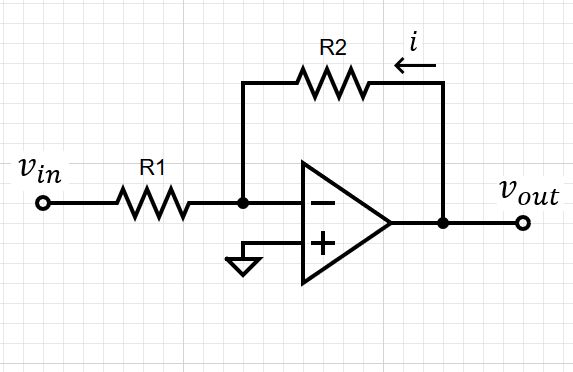top of page
Search
• Bob KØNR

# Operational Amplifiers: Basic Building Blocks

Operational amplifiers (commonly called op amps) are a ubiquitous building block for designing electronic circuits. Today, these devices are fabricated as small integrated circuits but the concept started long ago using vacuum tubes. Op amps are commonly used for DC and audio circuits but high-performance op amps can be used at radio frequencies.

## Ideal Operational Amplifier

To understand basic op amp functions, we use the concept of the Ideal Op Amp. The Ideal Op Amp is a voltage-controlled voltage source as shown in Figure 1, with these attributes:

1. Infinite gain (Av) and infinite bandwidth

2. Zero output impedance

3. Infinite input impedance (zero input current)Figure 1. The ideal op amp is a voltage-controlled voltage source with infinite input impedance and zero output impedance.

An important fourth attribute is usually included but it is only valid if there is negative feedback applied to the op amp:

4. Zero volts between the two inputs

These ideal op amp assumptions are quite amazing: infinite bandwidth and infinite gain? A key point is that for typical low frequency circuits, these assumptions hold quite well due to the excellent performance of common op amps. For more demanding applications, we may need to do a more careful analysis to understand how the circuit will perform. But for our purposes, we will just use the ideal assumptions.

The cool thing about op amps is that for many non-critical applications the op amp performance (gain, bandwidth, impedance, etc.) is so good compared to the circuit requirements that they really do act like ideal op amps. They are easy to design with and have become an essential building block for electronic systems.

In case you are wondering how this op amp gets its power, there are two power supply connections (positive and negative) for the device, often neglected when discussing the circuit design (but are absolutely essential when wiring up a real circuit). Typically, bipolar power is supplied, + 15 and - 15 volts, which supports a healthy signal swing.

## Non-Inverting Amplifier

The first common op amp configuration we will look at is the Non-Inverting Amplifier. (I always wonder why we don’t call this the “regular amplifier” configuration or maybe just “amplifier.”)Figure 2. The non-inverting amplifier uses two resistors to provide negative feedback to the op amp.

In this configuration, we see that we have feedback from the output back to the inverting input. This means that the two inputs will always have zero voltage across them. Because no current can flow into the inputs, the voltage present at the non-inverting input is determined by the voltage divider formed by R1 and R2.Rearranging to obtain the gain of the amplifier,Notice that the voltage gain of the circuit does not depend on the gain of the op amp. We are assuming that if the op amp gain is really big, then enough feedback will be applied to the non-inverting input to produce the desired function.

Let us check that assumption about the two op amp inputs having zero voltage between them. Suppose the non-inverting input is a few millivolts higher than the inverting input. The huge voltage gain of the op amp would cause the output to increase, which would feedback via the resistor divider to the inverting input. An increased voltage on the inverting input will cause the op amp output to decrease until both inputs have the same voltage. Thus, the high gain of the op amp plus negative feedback keeps the input voltages the same.

## Buffer Amplifier

A special case of the non-inverting amplifier is the buffer amplifier (also called unity-gain amplifier or voltage follower), having a voltage gain of one. This is equivalent to making R2 zero and R1 infinite in the non-inverting amplifier configuration. Again, negative feedback is applied such that the voltage between the op amp inputs is zero. This makes for a good buffer amplifier, with infinite impedance on the input and zero impedance on the output. Ideally, at least.Figure 3. The buffer amplifier provides infinite input impedance and zero output impedance.

## Inverting Amplifier

Another common op amp circuit is the inverting amplifier as shown in Figure 4. As the name implies, the output voltage is amplified with opposite polarity as the input.Figure 4. The inverting amplifier produces the negative value of the input, scaled by the ratio of the two resistors.

This circuit is analyzed by noting that both inputs of the op amp will be at zero volts. The non-inverting input is connected to ground and the inverting input will be driven to the same voltage via feedback through the resistors. We also note that current i flows through both resistors because no current enters the inverting input of the op amp.Rearranging to obtain the gain of the amplifier,The minus sign in the gain is important and must be considered in applying the circuit. In some situations, it may not matter, you just may need to amplify the input signal without regard to changes in polarity. In other cases, the polarity may be critical and your signal could end up being “upside down.”

We assumed that we have ideal op amps but we didn’t say anything about the resistors. The gain of these circuits will depend on the actual values of the resistors and therefore their tolerance.

## Summary

These common op amp circuits are useful for amplifying various analog signals. The ideal op amp model helps us understand how these circuits operate. For more detailed information on op amp circuits, refer to the excellent material in References 1 and 2.

References

1. Handbook of Operational Amplifier Applications, Bruce Carter and Thomas R. Brown, Texas Instruments, Sept 2016, http://www.ti.com/lit/an/sboa092b/sboa092b.pdf

2. Op Amps for Everyone, Ron Mancini, editor, August 2002, https://web.mit.edu/6.101/www/reference/op_amps_everyone.pdf

35 views

See All
bottom of page